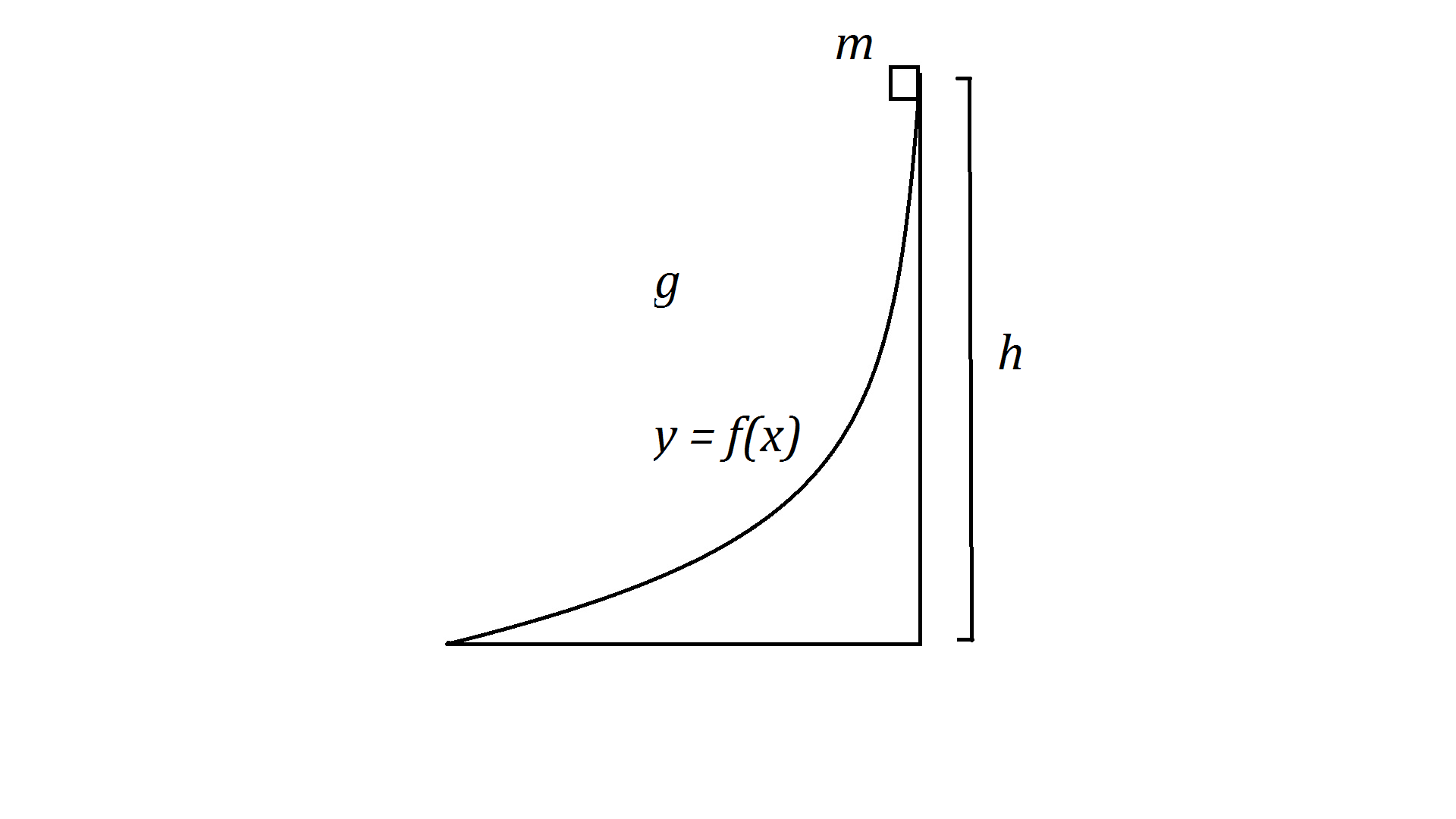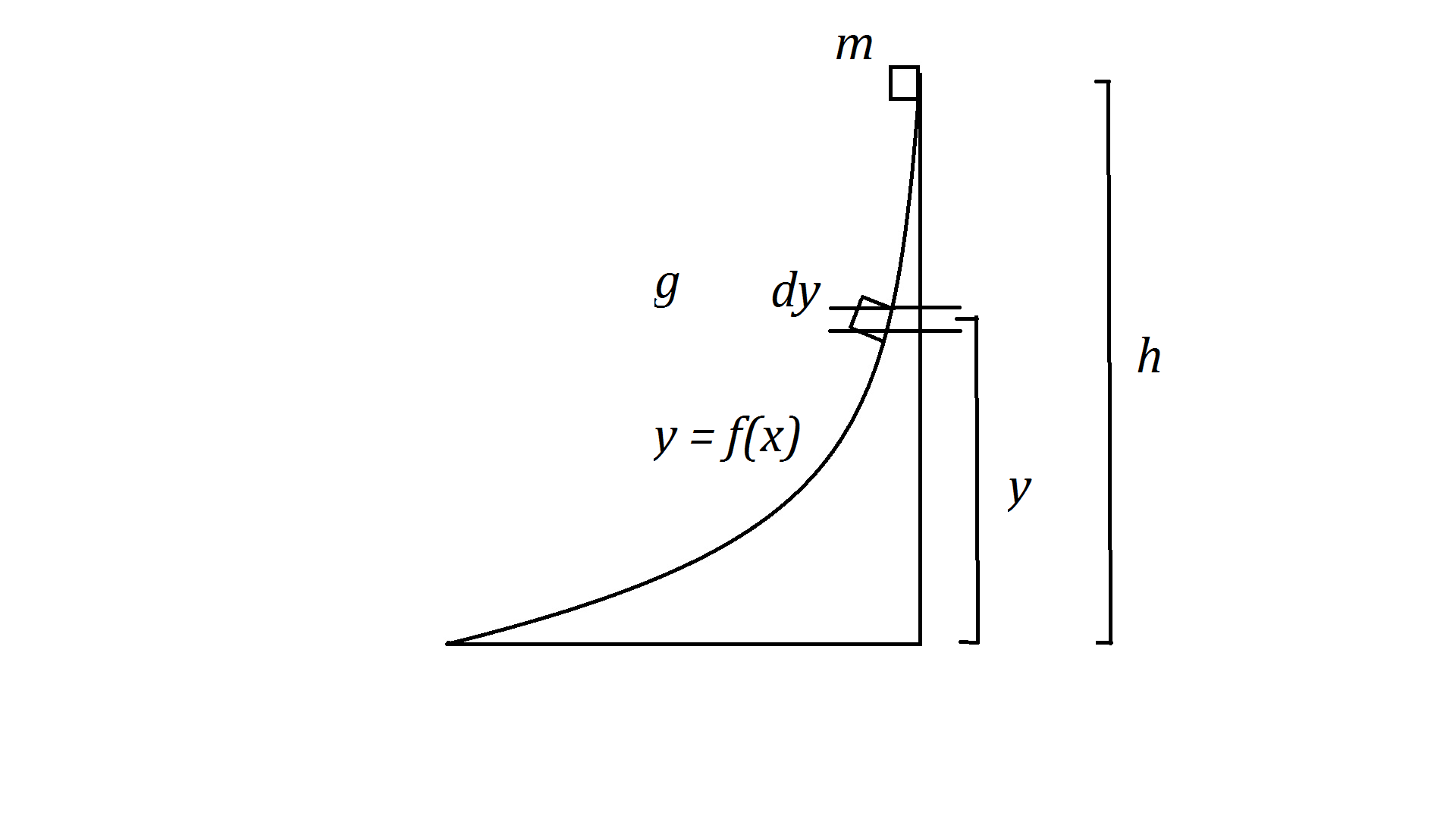# Period of a Pendulum

Back

Period of a Pendulum

1   ProblemConsider a block of mass$m$ released at the top of a frictionless quarter-circle incline of radius$R$. How much time$t$ does it take the block to travel to the bottom? Assume the dimensions of the box are much smaller than$R$ and that the block stays in contact with the surface of the incline at all times. The acceleration due to gravity is given by$g$.

You may make use of the integral$\alpha \equiv \int_0^{\pi/2} \frac{d\theta}{\sqrt{\cos\theta}} \approx 2.62206$

and use$\alpha$ in your solution.

2   Solution$t = \alpha\sqrt{\frac{R}{2g}}$

Derivation:

Look at the general case described below. Here we will use:$f(x) = R - \sqrt{R^2-x^2}$$f'(x) = \frac{x}{\sqrt{R^2-x^2}}$$f^{-1}(x) = \sqrt{2Rx - x^2}$$h = R$$t = \int_0^{f^{-1}(h)} \sqrt{\frac{1+f'^2(x)}{2g(h-f(x))}}dx$$f^{-1}(R) = R$$1 + f'^2(x) = \frac{R^2}{R^2-x^2}$$2g(h-f(x)) = 2g\sqrt{R^2-x^2}$$\frac{1 + f'^2(x)}{2g(h-f(x))} = \frac{R^2}{2g(R^2-x^2)^{3/2}}$$t = \int_0^R \sqrt{\frac{R^2}{2g(R^2-x^2)^{3/2}}}dx$$t = \frac{R}{\sqrt{2g}} \int_0^R \frac{dx}{(R^2-x^2)^{3/4}}$From the diagram,$\sin\theta = \frac{x}{R}$$dx = R\cos\theta d\theta$$R^2-x^2 = R^2\cos^2\theta$$x = 0, \theta = 0$$x = R, \theta = \pi/2$$t = \frac{R}{\sqrt{2g}} \int_0^{\pi/2} \frac{R\cos\theta d\theta}{(R\cos\theta)^{3/2}}$$t = \frac{R}{\sqrt{2g}} \int_0^{\pi/2} \frac{d\theta}{\sqrt{R\cos\theta}}$$t = \sqrt{\frac{R}{2g}} \int_0^{\pi/2} \frac{d\theta}{\sqrt{\cos\theta}}$

3   Pendulums

The motion of a block down a circular incline is identical to that of a simple pendulum. In this case, the period of a simple pendulum of varying amplitude can be derived by changing the height $h$ from which the block is released.$f^{-1}(h) = \sqrt{2Rh - h^2}$$2g(h-f(x)) = 2g(h - R + \sqrt{R^2-x^2})$$\frac{1 + f'^2(x)}{2g(h-f(x))} = \frac{R^2}{2g(R^2-x^2)(h-R+\sqrt{R^2-x^2})}$$t = \int_0^{\sqrt{2Rh - h^2}} \sqrt{\frac{R^2}{2g(R^2-x^2)(h-R+\sqrt{R^2-x^2})}}dx$$t = \frac{R}{\sqrt{2g}} \int_0^{\sqrt{2Rh - h^2}} \frac{dx}{\sqrt{(R^2-x^2)(h-R+\sqrt{R^2-x^2})}}$

Putting$h$ in terms of the amplitude$\theta$, it can be seen that$R - h = R\cos\theta$. Therefore,$h = R(1-\cos\theta)$.$\sqrt{2Rh - h^2} = R\sin\theta$$t = \frac{R}{\sqrt{2g}} \int_0^{R\sin\theta} \frac{dx}{\sqrt{(R^2-x^2)(\sqrt{R^2-x^2}-R\cos\theta)}}$

Period of a Pendulum of Amplitude$\theta_o$$T = \sqrt{\frac{8L}{g}} \int_0^{\theta_o} \frac{d\theta}{\sqrt{(\cos\theta-\cos\theta_o)}}$

Derivation:$T = 4t$$T = \frac{4R}{\sqrt{2g}} \int_0^{R\sin\theta_o} \frac{dx}{\sqrt{(R^2-x^2)(\sqrt{R^2-x^2}-R\cos\theta_o)}}$

Using the previous diagram,$x = 0, \theta = 0$$x = R\sin\theta_o, \theta = \theta_o$$T = \frac{4R}{\sqrt{2g}} \int_0^{\theta_o} \frac{R\cos\theta d\theta}{\sqrt{(R^2\cos^2\theta)(R\cos\theta-R\cos\theta_o)}}$$R=L$$T = \sqrt{\frac{8L}{g}} \int_0^{\theta_o} \frac{d\theta}{\sqrt{(\cos\theta-\cos\theta_o)}}$

4   General CaseConsider a block of mass$m$ released at a height$h$ on a frictionless, curved incline described by the function$y = f(x)$. How much time$t$ does it take the block to travel to the bottom? Assume the dimensions of the box are much smaller than$h$ and that the block stays in contact with the surface of the incline at all times. The acceleration due to gravity is given by$g$.$t = \int_0^{f^{-1}(h)} \sqrt{\frac{1+f'^2(x)}{2g(h-f(x))}}dx$

Derivation:When the block is located at a height$y$, its velocity can be derived from conservation of energy.$mg(h-y) = \frac{1}{2}mv^2$$v = \sqrt{2g(h-y)}$The time$dt$ it takes the block to travel down the incline is related to the distance$dl$ given by:$dl = \frac{v_o+v_f}{2}dt$

From the diagram:$dl = \sqrt{(dx)^2+(dy)^2}$

From above,$v_o$ is given by$\sqrt{2g(h-y)}$.$v_f$ is given by$\sqrt{2g(h-y+dy)}$. Ignoring first order differentials,$v_o = v_f = \sqrt{2g(h-y)}$.$\sqrt{(dx)^2+(dy)^2} = \sqrt{2g(h-y)}dt$$dy = f'(x) dx$$\sqrt{1 + f'^2(x)}dx = \sqrt{2g(h-f(x))}dt$$\sqrt{\frac{1+f'^2(x)}{2g(h-f(x))}}dx = dt$$\int_0^{f^{-1}(h)} \sqrt{\frac{1+f'^2(x)}{2g(h-f(x))}}dx = \int_0^t dt'$$t = \int_0^{f^{-1}(h)} \sqrt{\frac{1+f'^2(x)}{2g(h-f(x))}}dx$

Restrictions on$f(x)$:

We will require$f''(x) \geq 0$ in our interval so that the block’s velocity vector never points above the surface and hence the block will stay on the incline.

Simplifications:

The solution$t = \int_0^{f^{-1}(h)} \sqrt{\frac{1+f'^2(x)}{2g(h-f(x))}}dx$

gives the exact expression for the time taken, however no elementary antiderivative exists for most functions. We will make a practical approximation that will make it easy to evaulate the integral. If$f'(x) \gg 1$, we can rewrite$t$ as:$t \approx \int_0^{f^{-1}(h)} \sqrt{\frac{f'^2(x)}{2g(h-f(x))}}dx$$t \approx \frac{1}{\sqrt{2g}}\int_0^{f^{-1}(h)} \frac{f'(x) dx}{\sqrt{(h-f(x))}}$

We can make the u-substitution$u = h - f(x)$ and$du = - f'(x) dx$.$-\int \frac{du}{\sqrt{u}} = -2\sqrt{u}+C$$\int \frac{f'(x) dx}{\sqrt{(h-f(x))}} = -2\sqrt{h-f(x)} + C$$\int_0^{f^{-1}(h)} \frac{f'(x) dx}{\sqrt{(h-f(x))}} = -2\sqrt{h-f(f^{-1}(h))} + 2\sqrt{h-f(0)}$$t \approx \frac{2\sqrt{h-f(0)}}{\sqrt{2g}}$

If we have$f(0) = 0$, then$t \approx \sqrt{\frac{2h}{g}} = t_o$

which is the time it takes for the block to fall a height$h$ when released from rest. We can see that$t_o$ is sufficient in most cases.